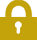##### Geometric Shapes #5###### Source: Listen In English

A simple drawing of geometric shapes including squares, circles, rectangles, triangles, vertical lines, horizonatal lines and diagonal lines.You can download the file [ HERE ].

###Directions

1. REVIEW the vocabulary.
2. LISTEN to the audio above.
3. DRAW the picture (on a piece of paper).
4. CHECK your drawing below.

###Vocabulary

If you don't know any of the words below, check the Illustrated Dictionary.

• a circle
• a rectangle
• a square
• a triangle
• a line
• horizontal
• vertical
• diagonal
• right-side up
• upside down
• side by side

###Grammar (prepositions)Directions: 1) Choose the determiner that you think best fits the sentence. 2) Listen again and check your answers.

• This is a picture (of) four figures - one (in) each corner (of) the page.
• (In) the top left corner (of) the page, draw a medium-sized square.
• On the left corner (of) the square, draw a small circle.
• Above the right corner (of) the square, draw another small circle about the same size.
• Inside the square, draw a diagonal line running (from) the top left corner (to) the bottom right corner.
• It does not touch the edges.
• (In) the bottom left corner (of) the page, draw an upside down triangle about the same size (as) the first square.
• Inside the triangle, draw a vertical line.
• The line does not touch the edges.
• (In) the top right corner (of) the page, draw two vertical rectangles side by side.
• In between the two rectangles are two small triangles.
• One triangle is (at) the top, and it is upside down.
• The other triangle is (at) the bottom, and it is right-side up.
• (In) the bottom right corner (of) the page, draw medium-sized circle about the same size (as) first square.
• Inside the circle, draw a horizontal line.
• The line does not touch the edges.

###ScriptThis is a picture of four figures - one in each corner of the page.

In the top left corner of the page, draw a medium-sized square.

On the left corner of the square, draw a small circle.

Above the right corner of the square, draw another small circle about the same size.

Inside the square, draw a diagonal line running from the top left corner to the bottom right corner.

It does not touch the edges.

In the bottom left corner of the page, draw an upside down triangle about the same size as the first square.

Inside the triangle, draw a vertical line.

The line does not touch the edges.

In the top right corner of the page, draw two vertical rectangles side by side.

In between the two rectangles are two small triangles.

One triangle is at the top, and it is upside down.

The other triangle is at the bottom, and it is right-side up.

In the bottom right corner of the page, draw medium-sized circle about the same size as first square.

Inside the circle, draw a horizontal line.

The line does not touch the edges.